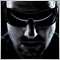# Looking for library which reflects iMA/iRSI etc. in MT5/MQL51220

Hi,

since the latest build of MT5, the background calculation of indicators, such as iMA() in MT5 seem to be changed. Result is, that it seems you have to deal with luck to get the values, especially when you request them within OnTimer() ... at it least this is what I suspect. When I drag such an (complex) indicator on a chart and switch between timeframes, sometimes it shows the results, sometimes not. Just like that, and this is useless. The only reason is to calculate the indicators manually by avoiding the iMA() function at all

Till now, I used some code which manages the indicator-handles that are returned by such functions, to avoid a creation of duplicate indicators. You can find a similar sample code below. It works without problems, also with the latest build. BUT ... as soon as your calculations in the indicator or expert get complex and need more time, the indicators aren´t calculated anymore, or maybe they are, but not always when you need the results. This method with this background calculation is absolutely horrible and a pretty bad idea. I don't understand it at all and I am sure I am not the only one. In a code, no matter if its and indicator or EA I need the data when I ask for it and not when there was some free time to calculate it. BarsCalulated(handle) ... really?

So my question is: Is there a class-library which contains all or most indicators and which delivers results on demand?

Thank you for your input guys.

```//+------------------------------------------------------------------+
//|                                                      MA_Test.mq5 |
//+------------------------------------------------------------------+
#property indicator_chart_window

//--- Struct for identification of MA indicators
struct S_MA_Handles
{
int            handle;
int            periods;
ENUM_MA_METHOD method;
};

//--- MA indicators data array
int            m_ma_cnt=0;
S_MA_Handles   m_ma[];

//+------------------------------------------------------------------+
//| Reset array of indicators (not used in test code)                |
//+------------------------------------------------------------------+
void      _MAReset()
{
for (int i=0;i<m_ma_cnt;i++)
::IndicatorRelease(m_ma[i].handle);
::ArrayFree(m_ma);
m_ma_cnt=0;
}

//+------------------------------------------------------------------+
//| Get handle for MA indicator                                      |
//+------------------------------------------------------------------+
#ifdef __MQL5__
int      _MAGetHandle(int periods,ENUM_MA_METHOD method)
{
int handle=INVALID_HANDLE;
for (int i=0;i<m_ma_cnt;i++)
{
if (m_ma[i].periods==periods && m_ma[i].method==method)
return m_ma[i].handle;
}

m_ma_cnt++;
::ArrayResize(m_ma,m_ma_cnt,4);
int index=m_ma_cnt-1;
m_ma[index].handle=iMA(::Symbol(),::Period(),periods,0,method,PRICE_CLOSE);
m_ma[index].periods=periods;
m_ma[index].method=method;
return m_ma[index].handle;
}
#endif

//+------------------------------------------------------------------+
//| Get MA value - main function                                     |
//+------------------------------------------------------------------+
double   MA(int periods, int shift, ENUM_MA_METHOD method)
{
//--- SMA negative value is interpreted as EMA
if (method==MODE_SMA && periods<0)
method=MODE_EMA;
periods=::MathAbs(periods);

//--- MT4
#ifdef __MQL4__
return iMA(::Symbol(),::Period(),periods,0,method,PRICE_CLOSE,shift);
#else
int handle=_MAGetHandle(periods,method);
//--- Debug
int c=::BarsCalculated(handle);
//-- Get value
int index=shift;
double value=__GetBuffer(handle,0,index,true);
return value;
#endif
}

#ifdef __MQL5__
double __GetBuffer(int handle,int index,int shift, bool wait=false)
{
if (handle==INVALID_HANDLE)
return 0;
static double buf;
::ResetLastError();
if (::CopyBuffer(handle,index,shift,1,buf)==1)
{
int e=GetLastError();
return(buf);
}

int e=::GetLastError();
if (!wait)
return 0;

::Sleep(50);
for (int i=0;i<100;i++)
{
if (::BarsCalculated(handle)>0)
break;
::Sleep(50);
}

if (::CopyBuffer(handle,index,shift,1,buf)>0)
return(buf);

return 0;
}
#endif

//+------------------------------------------------------------------+
//| Custom indicator initialization function                         |
//+------------------------------------------------------------------+
int OnInit()
{
EventSetMillisecondTimer(250);
//--- indicator buffers mapping

//---
return(INIT_SUCCEEDED);
}
//+------------------------------------------------------------------+
//| Custom indicator iteration function                              |
//+------------------------------------------------------------------+
int OnCalculate(const int rates_total,
const int prev_calculated,
const datetime &time[],
const double &open[],
const double &high[],
const double &low[],
const double &close[],
const long &tick_volume[],
const long &volume[],
{
//---

//--- return value of prev_calculated for next call
return(rates_total);
}
//+------------------------------------------------------------------+
//| Timer function                                                   |
//+------------------------------------------------------------------+
void OnTimer()
{

string result="";
for (int i=0;i<5;i++)
{
double v=MA(200,i,MODE_EMA);
result+=DoubleToString(v,SymbolInfoInteger(Symbol(),SYMBOL_DIGITS))+"/";
}
Print("EMA 200 values: ",result);

result="";
for (int i=0;i<5;i++)
{
double v=MA(20,i,MODE_SMA);
result+=DoubleToString(v,SymbolInfoInteger(Symbol(),SYMBOL_DIGITS))+"/";
}
Print("SMA 20 values: ",result);

//---

}
//+------------------------------------------------------------------+
```38519

I don't know what has changed or even if something has changed, not sure exactly. But you are totally right, working with complex indicators, multi-symbols and/or multi-timeframes is a complex matter if you want it to work in all cases (in normal conditions, when changing TF, on week-end when there is no ticks, when starting/restarting MT5, after computer sleeping, etc...). If you need to use several indicators (one calling an other, etc...) it just becomes a hell.

For example if you need to use this MA_Test indicator within an other indicator or EA with iCustom(), it will not work correctly because OnTimer() even is not triggered.

I managed it with a bunch of custom solutions, it works but with some mandatory compromises. It is still in testing as some "special cases" are not easy to manage. So, in summary, it's possible to do but far from easy, and I can't provide a comprehensive solution as my code is private. I sympathize.1220

It seems to be the same with CopyRates(). When you start the indicator and when you try to proceed all bars during OnTimer(), some rates are copied and some are not. If you wait some time ("some" ... really precise) you have access to all the bars. If not, it´s again a matter of luck. The developers can´t mean this for real.